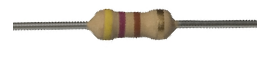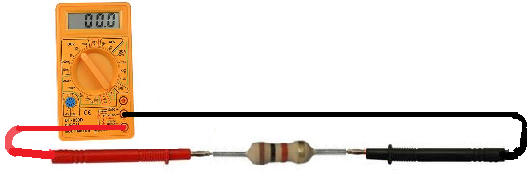﻿ How to Test a Resistor# How To Test a ResistorTo check to see whether a resistor is good or not, we need to only perform one test and this is to check the resistor's resistance value, using the ohmmeter of a multimeter.

### Test a Resistor with an Ohmmeter

Testing a Resistor with an ohmmeter is the best, easiest and most effective way to tell whether a resistor is good or not.

To set up for the check, we take the ohmmeter and place its probes across the leads of the resistor. The orientation doesn't matter, because resistance isn't polarized.The resistance that the ohmmeter reads should be close to the rated resistance of the resistor. For example, the following resistor above is a 1KΩ resistor with a tolerance rating of 5%. Therefore, the resistance of the resistor can vary between 950Ω and 1050Ω.

If the ohmmeter is reading in the value and tolerance range of the resistor, the resistor is good.

If the ohmmeter is reading (especially drastically) outside of this range, the resistor is defective and should be replaced.

### How to Test whether a Resistor is Open

If a resistor is reading a very high resistance, above its rated value, it is open. It is defective and, thus, should be replaced.

### How To Test whether a Resistor is Shorted

If a resistor is reading a very low resistance, near 0Ω, it's shorted internally. It is defective and, thus, should be replaced.

A resistance test is the only test that is needed to determine whether a resistor is good. If you want to examine more advanced features of a resistor, then additional tests may be necessary, but for all basic purposes, this test is sufficient for checking resistors.